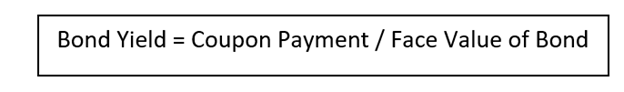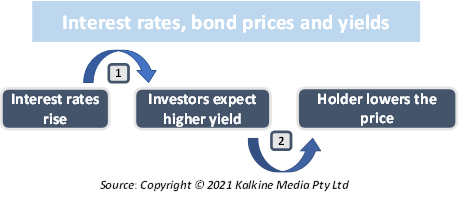Tue, September 21, 2021

# Bond Yield

• Updated on

## What is Bond Yield?

Bond yield refers to the annualized return received by an investor from a bond. Bond yield may not always be equal to the coupon rate as the current yield is dependent on the current market price. The current yield is also dependent on the coupon or interest rate.

When the price of the coupon differs from its face value, then the current yield is a more accurate depiction of the bond return than the actual bond yield. In its simplest form, bond yields can be calculated by dividing the coupon payment by the market price of the bond.

However, more intricate calculations of bond yields include time value of money and compounding interest payments.

## How do calculate the bond yield?

When an investor buys a bond, the issuer pays the investor equal amounts at regular intervals. At the date of maturity, the issuer pays the buyer the face value which is equal to the value at which the bond was initially bought.

Throughout the duration of the bond, the market price of the bond keeps changing, as it is traded in the market. The bond yield is calculated with the formula:Consider a bond priced at \$500, with coupon payments of \$50 paid every year. Therefore, the coupon rate, or the bond yield would be 50/500, which is 10%.

## Why do bond yields change?

It is important to note that if the buyer sells the bond in the market, it will not sell for its original face value of \$500 as the market price changes constantly. Thus, the bond can either be underpriced in the market or overpriced. If the bond sells for less than its face value, then it is at a discount and when it sells for more than its face value then it is selling at a premium.

The coupon amount offered to the buyer does not change. However, the discount and premium change the value of the yield offered to the bond holder.

## What are the factors affecting bond yields?

Prices and interest rates affect the bond yields. When the prices of a bond go up the yield decreases and vice versa. Thus, prices and yields have an inverse relationship. To keep coupon payments same, bond prices must adjust accordingly.

The interest rates also affect how yields move. Consider a bond offering a yield of 5%, with a coupon payment of \$100 paid annually with a 5-year maturity period. Now suppose the interest rates increase to 6%, any investor looking to buy the bond at par would be willing to receive a yield consistent with the market, i.e., 6%.However, in the current bond, the yield is fixed at 5%, so it is not possible to bring the yield at par for the new buyer. Therefore, the only way to sell the bond with a lower yield is to price it lower. Thus, as yields rise in the market, the price is lowered. The new price of the bond can be calculated via bond valuation.

On the contrary, if the interest rates had fallen in the market, the investor would be happy to buy the bond at the current price. The current investor is aware that the existing bond is priced lower for the yield it would offer however the existing bondholder would not want to sell it at a discount.

Thus, the existing bondholder would price the bond higher to not make a loss.

## How are bond yields different from interest rates?

Interest rates and bond yields are two terms used interchangeably. However, both are different. Interest rates and yields can sometimes move in opposite directions. Yields may sometimes be interpreted as the interest rates of bonds, however that is not an exact explanation of the term.

Interest rates are dependent on the market scenario. Thus, the interest rate charged on a deposit affects the interest amount received by the deposit holder. However, if the yields go up, the bond holder does not receive an increased coupon payment.

## What is meant by yield to maturity?

Yield to maturity refers to the yield that investors examine before buying a bond. Yield to maturity considers the following factors:

• Price: The higher the price of a bond the lower is its yield.
• Coupon rate: The higher the coupon rate, the higher is its yield. This happens because the bond pays a higher percentage of its face value in the form of payments.
• Time to maturity: The time remaining till maturity helps in finding out the compound interest one can earn on a bond upon reinvestment.
• The difference between face value and price: If the bondholder does not sell the bond then he would receive the face value back upon maturity. However, the face value may not always be the same as the price paid for the bond. This difference is captured by yield to maturity.

Yield to maturity has some variations, depending on the bonds that have embedded options. For instance, yield to call assumes that the bond will be called. This means that the bond is repurchased by the issuer before maturity. YTC is calculated with the assumption that the bond will be called as soon as possible.

Alternatively, yield to put is the same as YTC, with the only difference being that the holder can choose the price at which it wants to sell the bond. YTP is calculated on the assumption that it would be put back to the issuer as soon as possible.

Another variation includes yield to worst, which is calculated when a bond has multiple options. Any bond with both put and call provisions would use YTW based on the option terms that offer the lowest yield.

## How do yields affect the economy?

Higher bond yields are not taken as a good indication of the economic state. Rising bond yield raises the cost of capital for companies which ends up affecting their stock valuations. This means that stock markets are adversely affected due to rising yields.

Central banks may reduce liquidity support in case bond yields continue to rise. The government would conduct monetary contraction as it would become difficult to repay government debt as rates increase. Thus, if yields increase continually then it is often taken as an alarming signal and can hamper stock markets and be a sign of rising inflation in the future.